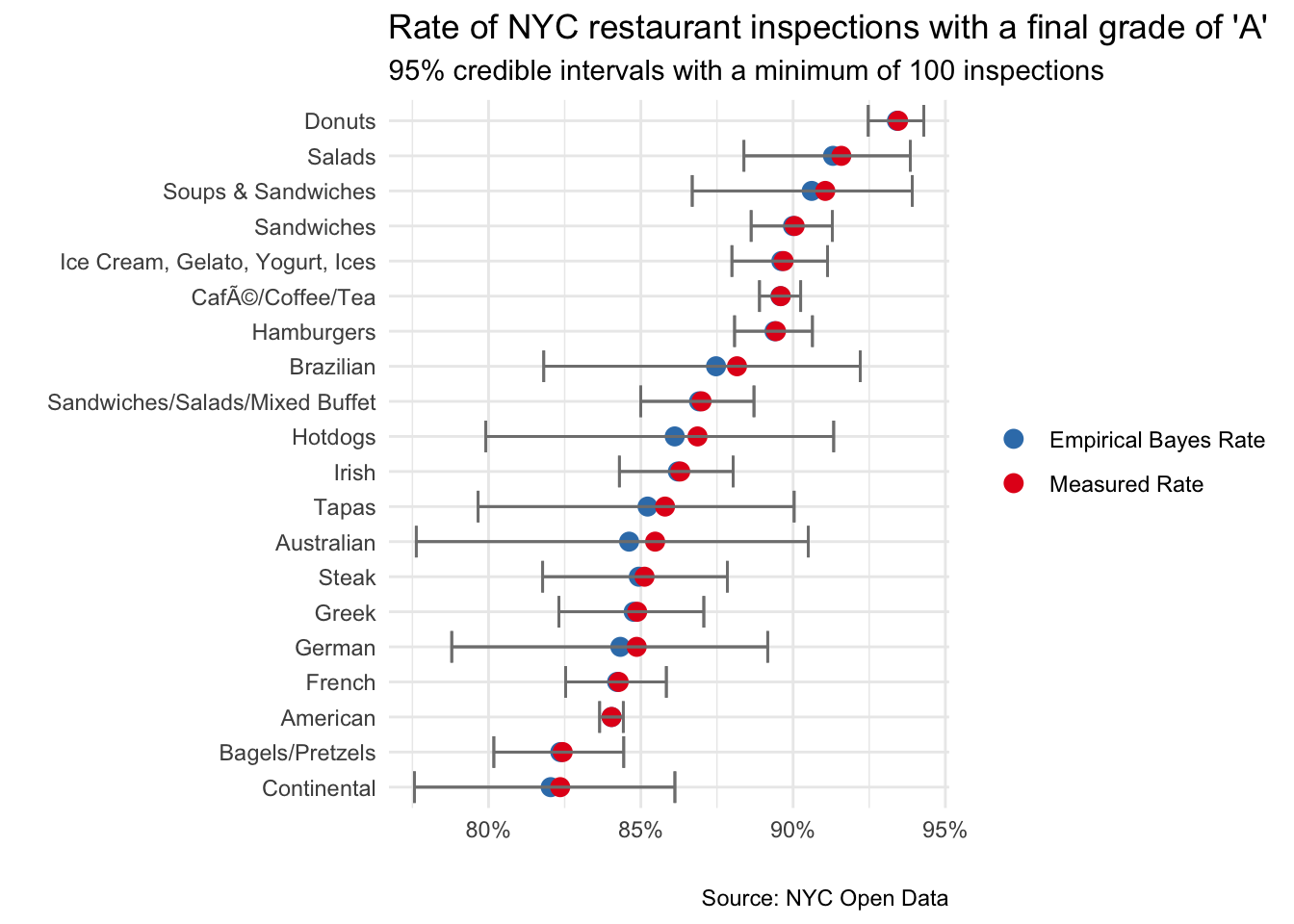Analyzing data for #tidytuesday week of 12/11/2018 (source)

``````# LOAD PACKAGES AND PARSE DATA
library(tidyverse)
library(scales)
library(RColorBrewer)
library(forcats)
library(lubridate)
library(ebbr)

nyc_restaurants <- nyc_restaurants_raw %>%
filter(inspection_date != '01/01/1900') ``````

### What is the rate of “A” inspection grades by cuisine type?

First step is to compute the relevant statistics

``````cuisine_grades <- nyc_restaurants %>%
na.omit() %>%
group_by(cuisine_description) %>%
mutate(total = sum(n),
pct_total = n/total) %>%
ungroup()``````

Next we apply empirical Bayesian estimation and filter the top 20 results

``````ebb_cuisine_grades <- cuisine_grades %>%
arrange(desc(.fitted)) %>%
filter(n >= 100) %>%
``````## Warning: `data_frame()` is deprecated as of tibble 1.1.0.
## This warning is displayed once every 8 hours.
## Call `lifecycle::last_warnings()` to see where this warning was generated.``````

Plot the graph to compare raw vs Bayesian shrinkage

``````ebb_cuisine_grades %>%
select(cuisine_description,
"Empirical Bayes Rate"=.fitted,
"Measured Rate"=.raw, .low, .high) %>%
gather(key, value, -cuisine_description, -.low, -.high) %>%
ggplot() +
geom_point(aes(reorder(cuisine_description, value), value, color = key),
size = 3) +
geom_errorbar(aes(ymin = .low, ymax = .high, x=cuisine_description),
color = "gray50") +
scale_y_continuous(labels = percent_format(round(1))) +
coord_flip() +
theme_minimal() +
labs(x = "",
y = "",
title = "Rate of NYC restaurant inspections with a final grade of \'A\'",
subtitle = "95% credible intervals with a minimum of 100 inspections",
caption = "Source: NYC Open Data") +
scale_color_brewer(palette = 'Set1',
direction = -1) +
theme(legend.title=element_blank())``````